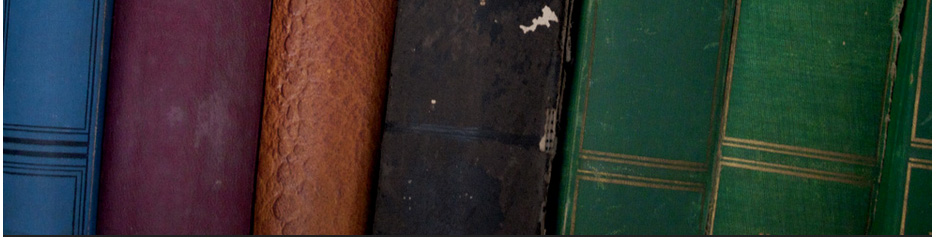## Primary education# Maths [video] for ks2

 ks20001 Partitioning Method (Addition of 4 Digits) – Key Stage 2 Maths Help ks20002 Partitioning Method (Addition of 3 Digits) – Key Stage 2 Maths Help ks20003 Primary Maths Subtraction ks20004 KS2 Maths SATs Revision – Q5 – Multiples and Factors ks20005 Empty Number Line Method (Subtraction) – Key Stage 2 Maths Help ks20006 Number line subtraction by Peter Weatherall ks20007 Dividing by 2 by Peter Weatherall ks20008 Measuring volume by Peter Weatherall ks20009 Alligator greater than by Peter Weatherall ks20010 How Negative Numbers Work ks20011 positive -negative- numbers ks20012 Understand and Learn the Rules of Positive and Negative Numbers ks20013 Dead Bunny’s Guide to Subtracting Integers ks20014 Add/Subtract Integers ks20015 How to: Convert Base 10 Integers to their Base 16 Value! ks20016 Converting From Base Ten into Other Bases ks20017 Converting from Different Bases Into Base Ten ks20018 Converting Fraction to Repeating or Terminating Decimals ks20019 Arithmetic Basics: Converting Decimals into Fractions ks20020 Converting fractions to decimals ks20021 Converting Fractions to Percentages ks10022 Change Fractions to Decimals to Percents ks20023 Great Lesson Ideas – Arithmetic Basics: Multiplying Decimals ks20024 Multiplying Decimals ks20025 SLEP Long Multiplication ks20026 Fractions & Proportions : How to Multiply With Decimal Points ks20027 Dividing Fractions k20028 Division of Fractions Part 1 ks20029 How to Find Percentages – YourTeacher.com – Math Help ks40030 Finding Percents

# Next page from number 31-60

Ermias Home

HAREP The Horn of Africa Research and Knowledge Exchange Platform © 2010-2012